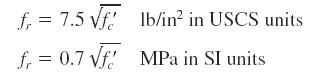Search

# Tensile Strength Of Concrete

Posted in Concrete Engineering |Email This Post |

The tensile strength of concrete is used in combined-stress design. In normal-weight, normal-density concrete the tensile strength can be found fromMore Entries :
• emmanuel abeiku inkum April 13, 2011 at 1:55 pm

im studying into the mechanical properties of cementitious material using high weight of sugar cane bagasse ash.
in the coarse of the trial mix, i was ordered by ma surperviser to achieve a standard slump of 65+or- 5mm.

• Rajesh kumar April 24, 2011 at 5:29 am

what is the tensile strength of cement concrete

• dinesh April 18, 2017 at 11:55 am

for example tensile strength of m20 grade concrete
tensile strength=0.7*(fck)^0.5
=0.7*(20)^0.5
tensile strength=3.130mpa (or)N/mmsquare

• prabu June 30, 2012 at 2:53 am

what is mean bytensile strength of steel?

• Mihir February 20, 2013 at 1:30 am

hey, if i need the design tensile strength, should i take it as (fr/1.5) or as 0.7(fck/1.5)^0.5…thanking in advance

• dale losser May 15, 2013 at 12:31 am

tensile equals strenght loads bend factors,radius etc.. ( titanium 6al 4v is around 158,000ksi……..)

• Awadh kumar pal October 29, 2015 at 3:02 am

tell me relationship b/w modulus of rupture & characteristic strength of concrete

• tatsam January 15, 2017 at 9:50 am

modulus of ruptre is tensile strength of concrete and characteristic strength is the max strength at which not more than 5 perecent of test result are expected to fail.